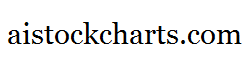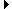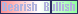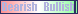Members AreaSign Up | Log In
 Free Level 2 QuotesStock AnalysisCorrelation Trading PlatformCorrelationsMembership Benefits

P1RANGE
 Lookup a different indicator.

 Indicator Usage Description Example p1range p1range[n] OPERATOR (INDICATOR or POSITIVE NUMBER) , where n is a positive integer >= 0. Returns the percentage change from the low to high price of day n where n=0 is the last trading day, n=1 is the previous day, etc. (p1range < 2)

Introduction:

The 'p1range' indicator is used in indicator formula construction to narrow the stock pre-screener results to include only those stocks that have a percentage change from the low to high price restricted to the parameters set for the p1range indicator.

Example:

The chart of Intel (INTC) below highlights days where the percentage change from the low to high stock price was less than 2%.

 Chart of Intel (INTC) highlighting days where the percentage change from the low to high stock price was less than 2%.

INDICATORS IN OUR DATABASE THAT INCLUDE THE P1RANGE INDICATOR...

Click here to view all indicators that include the indicator 'p1range' (sorted by AISCORE)

 (p1range < 2)AISCORE: 1524Indicator ID: 060419201556(close < sma) and (close > sma) and (vol > 1.05 * vol) and (p1range < 4)AISCORE: 1215Indicator ID: 060519102601(p4range > 1) and (p2change > 1) and (p1range > 4)AISCORE: 916Indicator ID: 060811174707(rsi > 52) and (p1range < 5) and (p1change < -2)AISCORE: 4611Indicator ID: 060811175100(p1change > 1) and (p4range < 5) and (p1range < 8)AISCORE: 1423Indicator ID: 060815224909 More ->